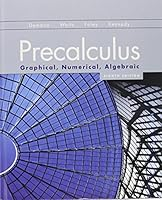# Precalculus: Graphical, Numerical, Algebraic (8th Edition)## Book Description

In , the authors encourage , numerical, and of functions as well as a focus on problem solving, conceptual understanding, and facility with technology.  They have created a book that is designed for instructors and written for students making this the most effective precalculus text available today.

CHAPTER 1 Functions and Graphs
CHAPTER 2 Polynomial, Power, and Functions
CHAPTER 3 Exponential, Logistic, and Logarithmic Functions
CHAPTER 4 Trigonometric Functions
CHAPTER 5 Analytic Trigonometry
CHAPTER 6 Applications of Trigonometry
CHAPTER 7 Systems and Matrices
CHAPTER 8 Analytic Geometry in Two and Three Dimensions
CHAPTER 9 Discrete Mathematics
CHAPTER 10 An Introduction to : Limits, Derivatives, and Integrals

APPENDIX A Algebra Review
APPENDIX B Key Formulas
APPENDIX C Logic